• 通过Gaver-Stehfest算法或任意函数及其参数执行拉普拉斯逆变换。 有关更多详细信息，请参见以下参考。 Villinger, H.，1985，使用 Gaver-Stehfest 拉普拉斯逆变换解决圆柱形地热问题，地球物理学，卷。 50 号10 页 ...matlab
• 您可以按照代码中给出的示例来构建您的二维二维拉普拉斯变换域中的函数，将其插入我们示例的位置，然后您就可以得到函数的二维拉普拉斯逆变换matlab
• 在这项工作中，我们给出了涉及线性因子乘积的第n个根的函数的拉普拉斯逆变换的四个结果。 为了找到拉普拉斯逆变换，我们考虑了第n个根的分支割和适当积分的区域，以避免出现分支点。 因此，该解决方案是针对积分的，...
• 拉普拉斯逆变换及matlab实现, 可以求取其极值点和零点
• 传递函数类的逆拉普拉斯变换计算器允许使用传递函数直接进行拉普拉斯变换由 N. Dincer Saygili 编码示例：gt = ilaplacetf(G) 其中G是传递函数类，gt是G的时域等效项matlab
• 为了找到执行拉普拉斯逆变换的稳定，准确和计算有效的方法，提出了一种新的双重变换方法。 为了验证和改进使用Gaver-Stehfest算法获得的反演解，对数字反演进行了直接拉普拉斯变换，以与原始函数进行比较。 数值直接...
• 使用MATLAB进行拉普拉斯逆变换
为什么要进行拉普拉斯逆变换
有些函数中复频域中求解很困难，我们需要把它变换到时域中，或者想看它在时域中有什么特性为了应付考试中的变态题目
函数名及使用步骤
使用syms 对变量进行定义使用ilaplace()进行拉普拉斯逆变换
示例
简单的幂函数 
octave:2> syms s;
octave:3> ilaplace(1/s^3)
ans = (sym)

2
t
──
2
  简单的指数函数  Octave的显示更=跟MATLAB不太一样
 octave:4> syms w;
octave:5> ilaplace(2/(w+s))
ans = (sym)

-t⋅re(w) - ⅈ⋅t⋅im(w)
2⋅ℯ
  三角函数 octave:7> ilaplace(s/(s^2+4))
ans = (sym) cos(2⋅t)

复杂函数的拉普拉斯逆变换
现实中有很多函数通过手算有很大的计算量，或者根本没有原函数，这个时候我们就只能通过MATLAB来求解

一个例子：我们把它输入到MATLAB中：
octave:8> F = (5-3*s)/(2+5*s)
F = (sym)

5 - 3⋅s
───────
5⋅s + 2

octave:9> f = ilaplace(F)
f = (sym)

-2⋅t
─────
⎛   s               ⎞      5
- 3⋅InverseLaplaceTransform⎜───────, s, t, None⎟ + ℯ
⎝5⋅s + 2            ⎠


上述结果也可以表示为：
ilaplace(F)
ans = -3/5*dirac(t)+31/25*exp(-2/5*t)


注意：
其中dirac(t)称为狄拉克函数，在自动控制和信号与系统中也叫单位脉冲函数

一个更复杂的例子：与上述步骤类似：
octave:11> F = (-s^2-9*s+4)/(s^2+s+2)
F = (sym)

2
- s  - 9⋅s + 4
──────────────
2
s  + s + 2

octave:12> f = ilaplace(F)
Waiting....!!! OUT OF TIME !!!

这里Octive已经崩溃了，可见这个原函数实在是太复杂了，我们这里就不展示了，浪费空间。。。。
配合ezplot()函数绘制逆变换后的图像
ezplot能够进行符号计算，不需要自变量的数据就能根据函数特性绘制图像，但通常要给自变量指定区间

例1求解原函数  syms s;
F = 1/(s+7).^2;
f = ilaplace(F);

 f =

t*exp(-7*t)
  绘制某个区间的图像  ezplot(f)
  图像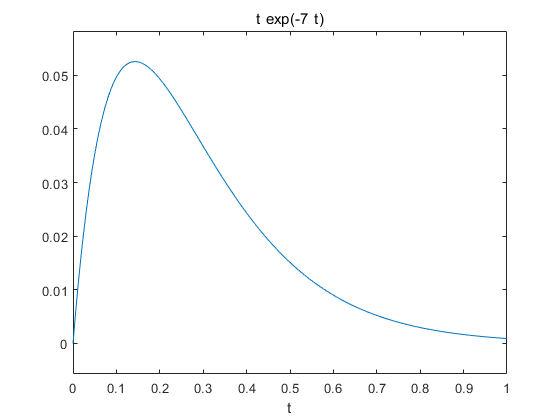例2与上述步骤类似，我们直接放代码及结果：
代码  syms s;
F = (2*s+3)/(((s+1).^2)*((s+3).^2));
f = ilaplace(F)
ezplot(f,[0 1])
 f =

exp(-t)/4 - exp(-3*t)/4 + (t*exp(-t))/4 - (3*t*exp(-3*t))/4
  图像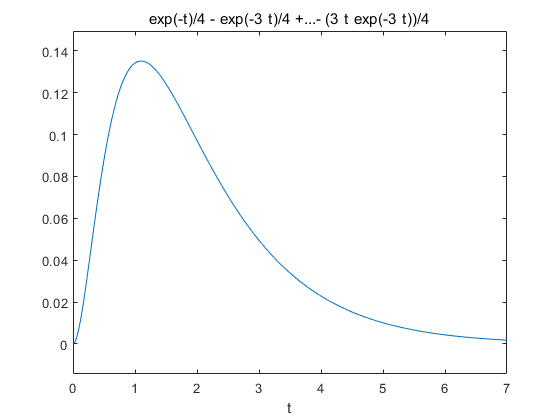展开全文matlab
• 我们根据拉普拉斯逆变换的定义式 去解太麻烦了，一般我们用部分分式展开法、查表法求拉普拉斯逆变换。 【 1. 查表法 】 例： 【 2. 部分分式展开法 】 1. F(s)有单极点（特征根为单根） 系数 Ki 的求解...


文章目录
【 1. 查表法 】【 2. 部分分式展开法 】1. F(s)有单极点（特征根为单根）2. F(s)有共轭单极点（特征根为共轭单根）

我们根据拉普拉斯逆变换的定义式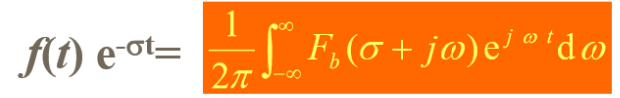去解太麻烦了，一般我们用部分分式展开法、查表法求拉普拉斯逆变换。

【 1. 查表法 】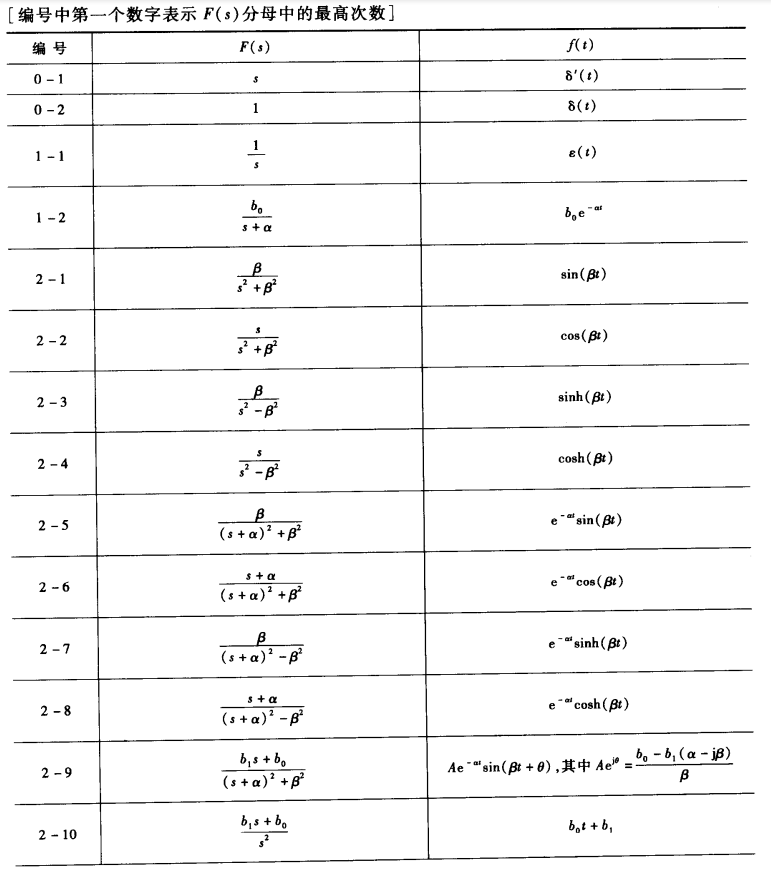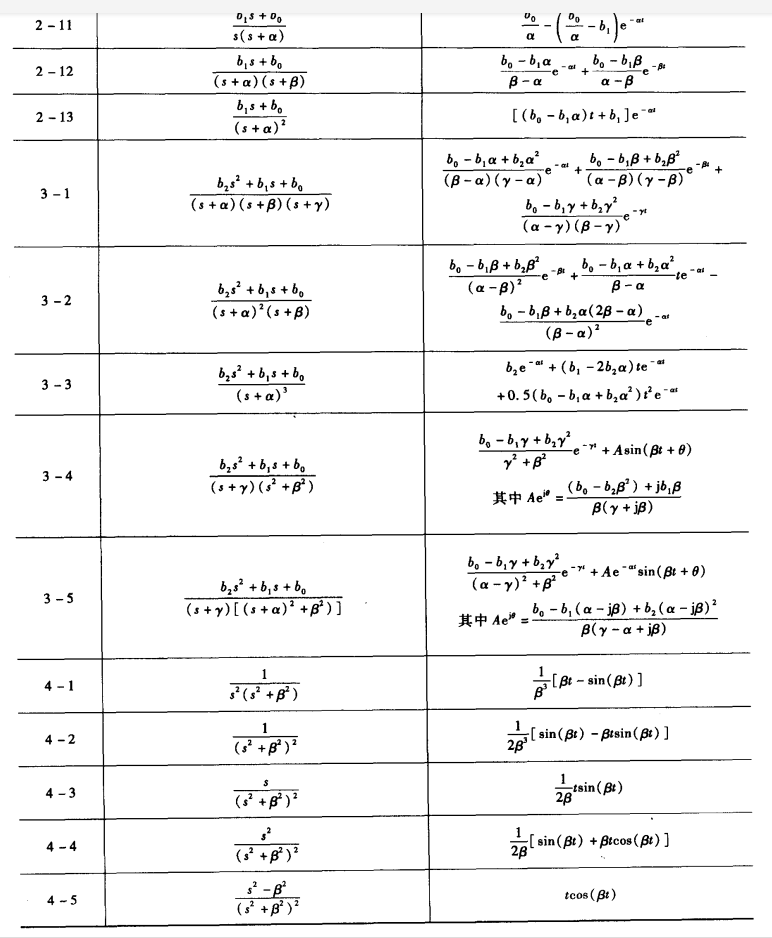例：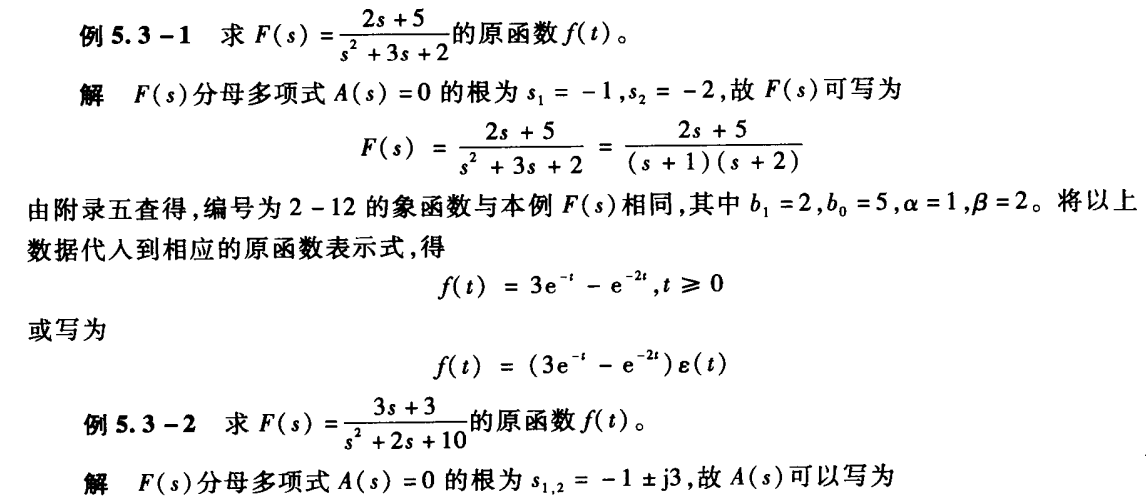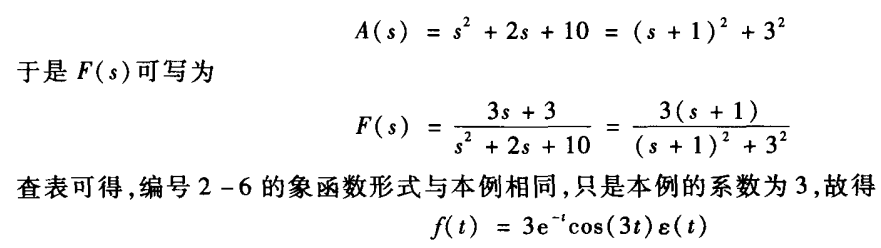【 2. 部分分式展开法 】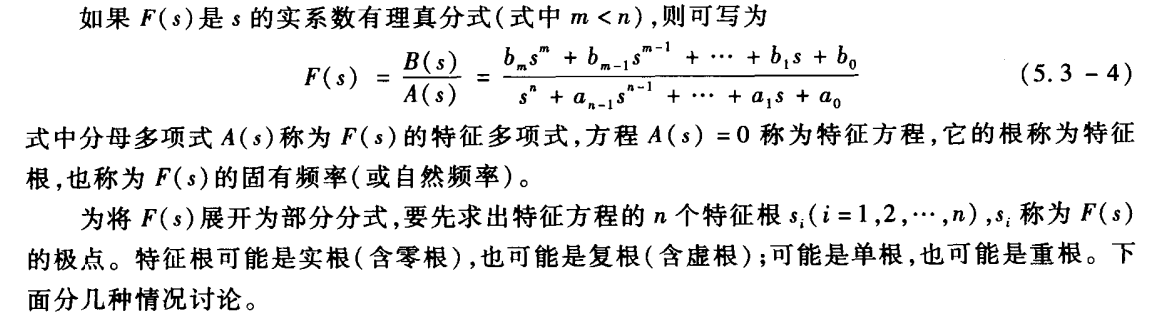1. F(s)有单极点（特征根为单根）系数 Ki 的求解：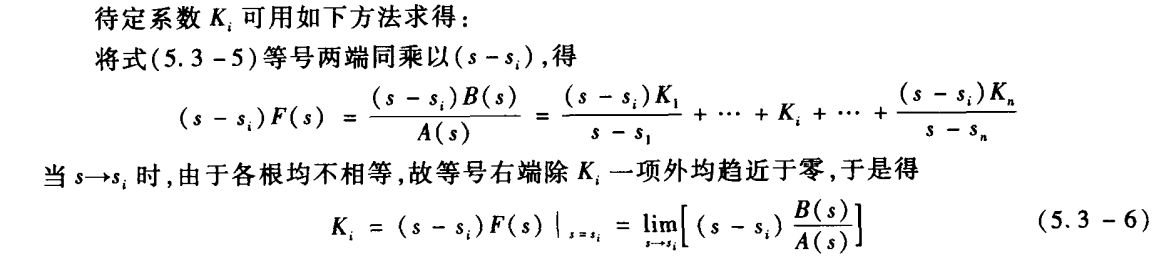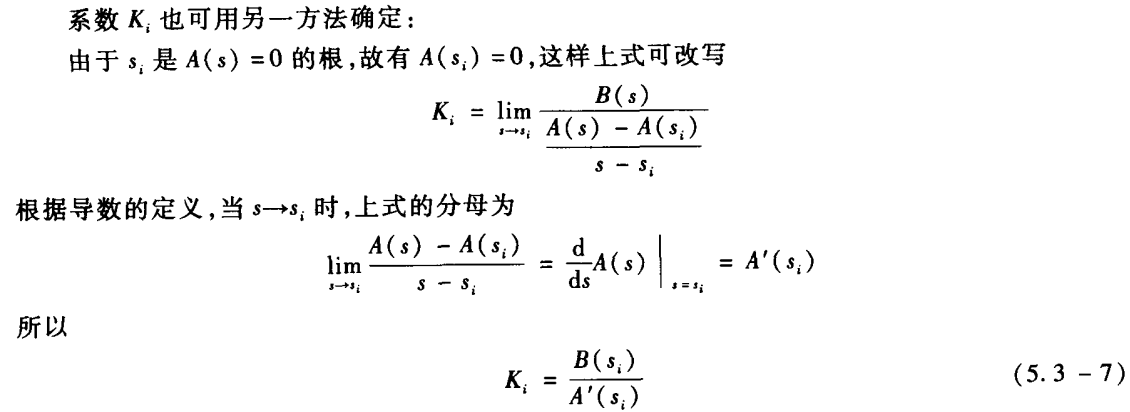2. F(s)有共轭单极点（特征根为共轭单根）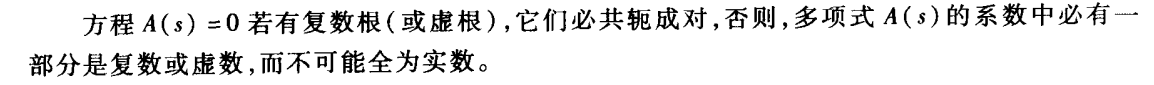例：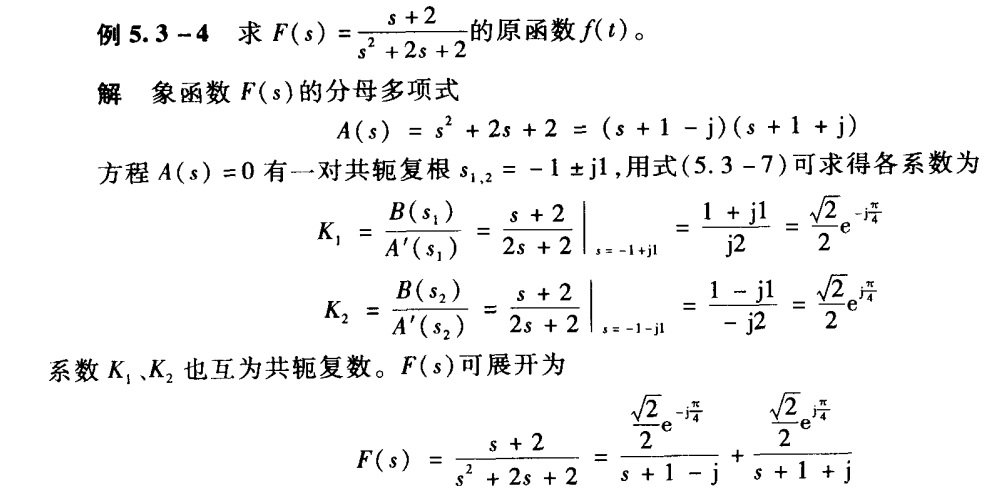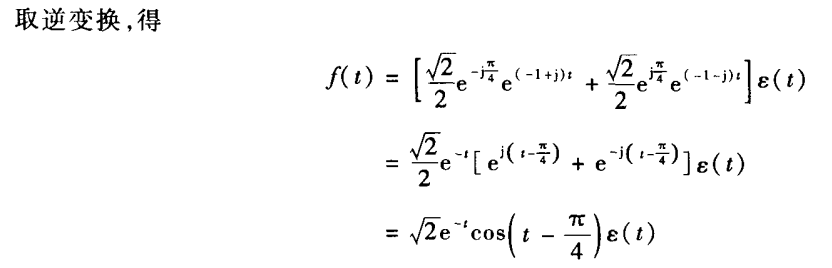展开全文• rilt 正则化拉普拉斯逆变换[g,yfit,cfg] = rilt(t,y,s,g0,alpha) 数组 g(s) 是数组 y(t) 的拉普拉斯逆变换，通过正则化最小二乘法计算。 该脚本是 S. Provencher CONTIN 程序的仿真，用 Fortran 编写。 请参阅...matlab
• 拉普拉斯逆变换

Author：AXYZdong 自动化专业 工科男 有一点思考，有一点想法，有一点理性！ CSDN@AXYZdong

文章目录
前言部分分式分解有理真分式的情形1、极点为实数，无重根2、包含共轭复数极点3、有多重极点
总结

前言
拉普拉斯逆变换求解方法：
（1）根据定义，复变函数积分（比较困难）
（2）部分分式分解（常用）
（3）留数定理
部分分式分解
若象函数

F

(

s

)

F(s)

是

s

s

的有理分式，可写为：

F

(

s

)

=

b

m

s

m

+

b

m

−

1

s

m

−

1

+

.

.

.

+

b

1

s

+

b

0

s

n

+

a

n

−

1

s

n

−

1

+

.

.

.

+

a

1

s

+

a

0

F(s)=\frac{b_ms^m+b_{m-1}s^{m-1}+...+b_1s+b_0}{s^n+a_{n-1}s^{n-1}+...+a_1s+a_0}

若

m

≥

n

m \ge n

（假分式），可用多项式除法将象函数

F

(

s

)

F(s)

分结尾有理多项式

P

(

s

)

P(s)

与有理真分式之和。

F

(

s

)

=

P

(

s

)

+

B

0

(

s

)

A

(

s

)

F(s)=P(s)+\frac{B_0(s)}{A(s)}

有理真分式的情形
若

F

(

s

)

F(s)

是

s

s

实系数有理真分式（

m

<

n

m<n

），则可写为：

F

(

s

)

=

B

(

s

)

A

(

s

)

=

b

m

s

m

+

b

m

−

1

s

m

−

1

+

.

.

.

+

b

1

s

+

b

0

s

n

+

a

n

−

1

s

n

−

1

+

.

.

.

+

a

1

s

+

a

0

F(s)=\frac{B (s)}{A(s)}=\frac{b_ms^m+b_{m-1}s^{m-1}+...+b_1s+b_0}{s^n+a_{n-1}s^{n-1}+...+a_1s+a_0}

A

(

s

)

A(s)

称为特征多项式，方程

A

(

s

)

=

0

A(s)=0

称为特征方程，它的根称为特征根，也称为

F

(

s

)

F(s)

的固有频率。

n

n

个特征根

p

i

p_i

称为

F

(

s

)

F(s)

的极点。
1、极点为实数，无重根
例：

F

(

s

)

=

2

s

+

1

s

(

s

+

2

)

(

s

+

3

)

F(s)=\frac{2s+1}{s(s+2)(s+3)}

解：令：

F

(

s

)

=

k

1

s

+

k

2

s

+

2

+

k

3

s

+

3

F(s)=\frac{k_1}{s}+\frac{k_2}{s+2}+\frac{k_3}{s+3}

k

1

=

s

F

(

s

)

∣

s

=

0

=

1

/

6

,

k

2

=

(

s

+

2

)

F

(

s

)

∣

s

=

−

2

=

3

/

2

k

3

=

(

s

+

3

F

(

s

)

∣

s

=

−

3

=

−

5

/

3

k_1=sF(s)|_{s=0}=1/6,\\k_2=(s+2)F(s)|_{s=-2}=3/2\\k_3=(s+3F(s)|_{s=-3}=-5/3

故：

F

(

s

)

=

1

6

s

+

3

2

(

s

+

2

)

+

−

5

3

(

s

+

3

)

F(s)=\frac{ 1}{6s}+\frac{3}{2(s+2)}+\frac{-5}{3(s+3)}

F

(

s

)

F(s)

拉式反变换为

f

(

t

)

=

(

1

6

+

3

2

e

−

2

t

−

5

3

e

−

3

t

)

ϵ

(

t

)

f(t)=( \frac{ 1}{6}+\frac{3}{2}e^{-2t}-\frac{5}{3}e^{-3t})\epsilon(t)

2、包含共轭复数极点
例：

F

(

s

)

=

s

2

+

3

(

s

+

2

)

(

s

2

+

2

s

+

5

)

F(s)=\frac{s^2+3}{ (s+2)(s^2+2s+5)}

解：

F

(

s

)

=

s

2

+

3

(

s

+

1

+

j

2

)

(

s

+

1

−

j

2

)

(

s

+

2

)

F(s)=\frac{s^2+3}{(s+1+j2)(s+1-j2)(s+2)}

令：

F

(

s

)

=

k

1

s

+

1

−

j

2

+

k

2

s

+

1

+

j

2

+

k

3

s

+

2

F(s)=\frac{k_1}{s+1-j2}+\frac{k_2}{s+1+j2}+\frac{k_3}{s+2}

p

1

,

2

=

−

α

±

j

β

,

(

α

=

1

,

β

=

2

)

p_{1,2}=-\alpha\pm j\beta,(\alpha=1,\beta=2)

k

1

=

(

s

+

1

−

j

2

)

F

(

s

)

∣

s

=

−

1

+

j

2

=

−

1

+

j

2

5

k_1=(s+1-j2)F(s)|_{s={-1+j2}}=\frac{-1+j2}{5}

即

：

k

1

,

2

=

A

±

j

B

,

(

A

=

−

1

5

,

B

=

2

5

)

即：k_{1,2}=A\pm jB,(A=-\frac{1}{5},B=\frac{2}{5})

k

1

=

(

s

+

2

)

F

(

s

)

∣

s

=

−

2

=

7

5

k_1=(s+2)F(s)|_{s=-2}=\frac{7}{5}

故：

F

(

s

)

=

−

1

+

j

2

5

s

+

1

−

j

2

+

−

1

−

j

2

5

s

+

1

+

j

2

+

7

5

s

+

2

F(s)=\frac{\frac{-1+j2}{5}}{s+1-j2}+\frac{\frac{-1-j2}{5}}{s+1+j2}+\frac{\frac{7}{5}}{s+2}

F

(

s

)

F(s)

拉式反变换为

f

(

t

)

=

{

2

e

−

t

[

−

1

5

cos

⁡

(

2

t

)

−

2

5

sin

⁡

(

2

t

)

]

+

7

5

e

−

2

t

}

ϵ

(

t

)

f(t)=\lbrace 2e^{-t}[-\frac{1}{5}\cos(2t)-\frac{2}{5}\sin(2t)]+\frac{7}{5}e^{-2t}\rbrace \epsilon(t)

3、有多重极点
例：

F

(

s

)

=

s

−

2

s

(

s

−

1

)

2

F(s)=\frac{s-2}{s(s-1)^2}

解：令：

F

(

s

)

=

k

11

(

s

−

1

)

2

+

k

12

(

s

−

1

)

+

k

2

s

F(s)=\frac{k_{11}}{(s-1)^2}+\frac{k_{12}}{(s-1) }+\frac{k_2}{s}

令：

F

1

(

s

)

=

(

s

−

1

)

2

F

(

s

)

=

s

−

2

s

F_1(s)=(s-1)^2F(s)=\frac{s-2}{s}

k

11

=

F

1

(

s

)

∣

s

=

1

=

−

1

,

k

12

=

d

d

s

F

1

(

s

)

=

s

−

(

s

−

2

)

s

2

∣

s

=

1

=

2

,

k

2

=

s

F

(

s

)

∣

s

=

0

=

−

2

k_{11}=F_1(s)|_{s=1}=-1,k_{12}=\frac{d}{ds}F_1(s)=\frac{s-(s-2)}{s^2}|_{s=1}=2,k_2=sF(s)|_{s=0}=-2

故：

F

(

s

)

=

−

1

(

s

−

1

)

2

+

2

(

s

−

1

)

+

−

2

s

F(s)=\frac{-1}{(s-1)^2}+\frac{2}{(s-1) }+\frac{-2}{s}

F

(

s

)

F(s)

拉式反变换为

f

(

t

)

=

(

−

t

e

t

+

2

e

t

−

2

)

ϵ

(

t

)

f(t)=(-te^t+2e^t-2)\epsilon(t)

总结
部分分式分解还是比较常用的，注意分解步骤，计算时仔细一点。常用的拉氏变换要记得。

「你可能还想看」系列文章： 【信号与系统】笔记合集，你确定不收藏吗？我已经收藏了   看完就赞，养成习惯！！！^ _ ^ ❤️ ❤️ ❤️ 码字不易，大家的支持就是我坚持下去的动力。点赞后不要忘了关注我哦！
展开全文信号处理
• 这类问题在分数电路设计中经常出现，该函数可以有效地计算形式为 1/(s^u(s^va)) 的函数的拉勒斯变换，其中 u 和 v 可能是分数的。 在此过程中使用了分数积分的“Riemman-Louivelle”定义。matlab
• 利用MATLAB实现拉普拉斯变换及其逆变换PAGEPAGE 7信号与系统实践报告有MATLAB实现连续时间周期函数学 院：通信与电子信息工程学院 班 级：电子042班 姓 名：李瑞改 学 号：2004023082 指导教师：朱恒军 秦月2006年10...

利用MATLAB实现拉普拉斯变换及其逆变换
PAGE
PAGE 7
信号与系统实践报告
有MATLAB实现连续时间周期函数
学 院：通信与电子信息工程学院 班 级：电子042班 姓 名：李瑞改 学 号：2004023082 指导教师：朱恒军 秦月
2006年10月19日
摘要 ：本例的CTFShchsym.m函数文件有一定的通用性，用户只需编写好子函数time_fun_即可，但要注意，该函数是用符号表达式写成的。若要画出时间函数图形，用户需要另外编写一个子函数 y=time_fun_e(t)。因为在 MATLAB中，只定义了单位阶跃信号 Heavisid作为一个符号对象，而不能把 Heaviside 看作 MATLAB 的函数加以调用。 同理，在信号与系统中，另一个十分重要的函数——单位脉冲函数 Dirac(t)。它的使用方法可参照 Heaviside 进行。 最后给出的数值是由完全准确解取 32位有效数字后的简洁表示。 关键字：单位阶跃信号 MATLAB 连续信号 脉冲宽度
目的: 1. 函数文件 CTFStpshsym.m编写源程序 2. 有MATLAB实现连续时间周期函数
一：函数 step()将绘出连续系统的阶跃响应函数 step()将绘出由向量 a 和 b 表示的连续系统的阶跃响应g(t)在指定时间范围内的波形图，并能求出其数值解。和 impulse()函数一样，step()函数也有如下四种调用格式： (1) step(b,a) (2) step(b,a,t) (3) step(b,a,t1:p:t2) (4) y=step(b,a,t1:p:t2) 上述调用格式的功能和 impulse()函数完全相同，所不同的是命令绘制的是系统的阶跃响应g(t)的曲线而不冲激响应h(t)的曲线。对上例，若执行命令 step(b,a) 则绘制的系统阶跃响应时域波形如图所示。
连续系统的冲激响应 连续系统的阶跃响应
二： 函数文件 CTFStpshsym.m编写源程序 编写函数文件 CTFStpshsym.m [CTFStpshsym.m] function [A_sym,B_sym]= CTFStpshsym % 采用符号计算求[0,T]内时间函数的三角级数展开系数，并绘制其双边频谱。 % 函数的输出为数值量 % Nn 输出数据的准确位数 % A_sym 第 1元素是直流项，其后元素依次是 1,2,3...次谐波 cos项展开系数 % B_sym 第 2,3,4,...元素依次是 1,2,3...次谐波 sin项展开系数 % T T=m*tao， 信号周期 % Nf 谐波的阶数 % Nn 输出数据的准确位数 % m (m=T/tao)周期与脉冲宽度之比,如 m=4,8,16,100等 % tao 脉宽:tao=T/m syms t n y if nargin<3;Nf=input('pleas Input 所需展开的最高谐波次数:Nf=');end T=input('pleas Input 信号的周期 T='); if nargin<5;Nn=32;end y=time_fun_s(t); A0=2*int(y,t,0,T)/T; As=int(2*y*cos(2*pi*n*t/T)/T,t,0,T); Bs=int(2*y*sin(2*pi*n*t/T)/T,t,0,T); A_sym(1)=double(vpa(A0,Nn)); for k=1:Nf A_sym(k+1)=double(vpa(subs(As,n,k),Nn)); B_sym(k+1)=double(vpa(subs(Bs,n,k),Nn)); end if nargout==0 S1=fliplr(A_sym) %对 A_sym阵左右对称交换 S1(1,k+1)=A_sym(1) %A_sym的 1*k阵扩展为 1*(k+1)阵 S2=fliplr(1/2*S1) %对扩展后的 S1阵左右对称交换回原位置 S3=fliplr(1/2*B_sym) %对 B_sym阵左右对称交换 S3(1,k+1)=0 %B_sym的 1*k阵扩展为 1*(k+1)阵 S4=fliplr(S3) %对扩展后的 S3阵左右对称交换回原位置 S5=S2-i*S4; % 用三角函数展开系数 A、B值合成付里叶指数系数 S6=

展开全文• Laplace Inverse Transformation (LIT) L(1πte−a2/4t)=e−ass,&nbsp;&nbsp;a&amp;gt;0\mathcal{L}(\frac{1}{\sqrt{\pi t}}\text{e}^{-a^2/4t})=\frac{\text{e}^{-a\sqrt{s}}}{\sqrt{s}},~~ a&...
• def fs(s): return 1/((s+0.2)*(1+exp(-(s+0.2))))python
• matlab拉普拉斯变换和逆变换,通过具体编程掌握应用。包括matlab拉普拉斯变换和逆变换前、matlab拉普拉斯变换和逆变换中、matlab拉普拉斯变换和逆变换后。matlab matlab安装教程 matlab下载 matlab图像处理...